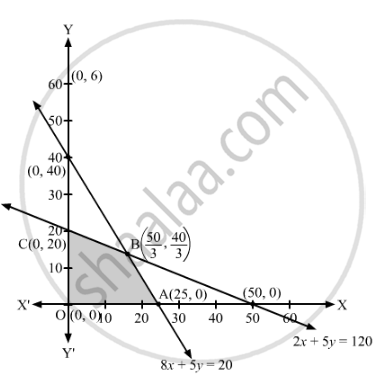# If a Young Man Drives His Vehicle at 25 Km/Hr, He Has to Spend ₹2 per Km on Petrol. If He Drives It at a Faster Speed of 40 Km/Hr, the Petrol Cost Increases to ₹5 per Km. - Mathematics

Sum

If a young man drives his vehicle at 25 km/hr, he has to spend ₹2 per km on petrol. If he drives it at a faster speed of 40 km/hr, the petrol cost increases to ₹5 per km. He has ₹100 to spend on petrol and travel within one hour. Express this as an LPP and solve the same.

#### Solution

Let us assume that the man travels x km when the speed is 25 km/hour and y km when the speed is 40 km/hour.

Thus, the total distance travelled is (x + y) km.

Now, it is given that the man has Rs 100 to spend on petrol.

Total cost of petrol = 2x + 5y ≤ 100

Now, time taken to travel x km = $\frac{x}{25}$  h Time taken to travel y km = $\frac{y}{40}$ h  Now, it is given that the maximum time is 1 hour. So,

$\frac{x}{25} + \frac{y}{40} \leq 1$
$\Rightarrow 8x + 5y \leq 200$
Thus, the given linear programming problem is

Maximise Z = x + y

subject to the constraints

2x + 5y ≤ 100

8x + 5y ≤ 200

x ≥ 0, y ≥ 0

The feasible region determined by the given constraints can be diagrammatically represented as,The coordinates of the corner points of the feasible region are O(0, 0), A(25, 0), B $\left( \frac{50}{3}, \frac{40}{3} \right)$ and  C(0, 20).

The value of the objective function at these points are given in the following table.

 Corner Points Z = x + y (0, 0) 0 + 0 = 0 (25, 0) 25 + 0 = 25 $\left( \frac{50}{3}, \frac{40}{3} \right)$ $\frac{50}{3} + \frac{40}{3} = 30$ (0, 20) 0 + 20 = 20

So, the maximum value of Z is 30 at $x = \frac{50}{3}, y = \frac{40}{3}$

Thus, the maximum distance that the man can travel in one hour is 30 km.

Hence, the distance travelled by the man at the speed of 25 km/hour is $\frac{50}{3}$  km, and the distance travelled by him at the speed of 40 km/hour is $\frac{40}{3}$ Km.

Concept: Graphical Method of Solving Linear Programming Problems
Is there an error in this question or solution?

#### APPEARS IN

RD Sharma Class 12 Maths
Chapter 30 Linear programming
Exercise 30.4 | Q 36 | Page 55